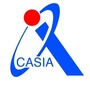0+0+0+0+0+0+0+0+0+0+0+0+0+0+0+0+0+0+0+0+0+0+0+0+0+0+0+0+0+0+Let \$vc(G)\$, \$fvs(G)\$ and \$oct(G)\$, respectively, denote the size of a minimum vertex cover, minimum feedback vertex set and minimum odd cycle transversal in a graph \$G\$. One can ask, when looking for these sets in a graph, how much bigger might they be if we require that they are independent; that is, what is the price of independence? If \$G\$ has a vertex cover, feedback vertex set or odd cycle transversal that is an independent set, then we let \$ivc(G)\$, \$ifvs(G)\$ or \$ioct(G)\$, respectively, denote the minimum size of such a set. Similar to a recent study on the price of connectivity (Hartinger et al. EuJC 2016), we investigate for which graphs \$H\$ the values of \$ivc(G)\$, \$ifvs(G)\$ and \$ioct(G)\$ are bounded in terms of \$vc(G)\$, \$fvs(G)\$ and \$oct(G)\$, respectively, when the graph \$G\$ belongs to the class of \$H\$-free graphs. We find complete classifications for vertex cover and feedback vertex set and an almost complete classification for odd cycle transversal (subject to three non-equivalent open cases). We also investigate for which graphs \$H\$ the values of \$ivc(G)\$, \$ifvs(G)\$ and \$ioct(G)\$ are equal to \$vc(G)\$, \$fvs(G)\$ and \$oct(G)\$, respectively, when the graph \$G\$ belongs to the class of \$H\$-free graphs. We find a complete classification for vertex cover and almost complete classifications for feedback vertex set (subject to one open case) and odd cycle transversal (subject to three open cases).0+0+0+下载预览0+1+0+0+0+0+0+0+0+0+0+0+
Top